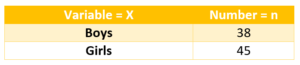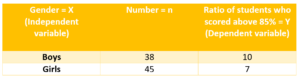When it comes to the level of analysis in statistics, there are three different analysis techniques that exist. These are –

• Univariate analysis
• Bivariate analysis
• Multivariate analysis

The selection of the data analysis technique is dependent the number of variables, types of data and focus of the statistical inquiry. The following section describes the three different levels of data analysis –

Univariate analysis

Univariate analysis is the most basic form of statistical data analysis technique. When the data contains only one variable and doesn’t deal with a causes or effect relationships then a Univariate analysis technique is used. For instance, in a survey of a class room, the researcher may be looking to count the number of boys and girls. In this instance, the data would simply reflect the number, i.e. a single variable and its quantity as per the below table. The key objective of Univariate analysis is to simply describe the data to find patterns within the data. This is be done by looking into the mean, median, mode, dispersion, variance, range, standard deviation etc.How Univariate analysis is conducted

Univariate analysis is conducted through several ways which are mostly descriptive in nature

• Frequency Distribution Tables
• Histograms
• Frequency Polygons
• Pie Charts
• Bar Charts

Bivariate analysis

Bivariate analysis is slightly more analytical than Univariate analysis. When the data set contains two variables and researchers aim to undertake comparisons between the two data set then Bivariate analysis is the right type of analysis technique. For example – in a survey of a classroom, the researcher may be looking to analysis the ratio of students who scored above 85% corresponding to their genders. In this case, there are two variables – gender = X (independent variable) and result = Y (dependent variable). A Bivariate analysis is will measure the correlations between the two variables as shown the table below.How Bivariate analysis is conducted

1. Correlation coefficients

Correlations is a statistical association technique where strength of relationship between two variables are observed. It shows the strength as strong or weak correlations and are rated on a scale of –1 to 1, where 1 is a perfect direct correlation, –1 is a perfect inverse correlation, and 0 is no correlation.

1. Regression analysis

Regression analysis is used for estimating the relationships between two different variables. It includes techniques for modelling and analysing several variables, when the focus is on the relationship between a dependent variable and one or more independent variables. It helps to understand how the value of the dependent variable changes when any one of the independent variables is changed. Regression analysis is used for advanced data modelling purposes like prediction and forecasting. There are a range of different regression techniques used depending on the nature of variable and the type of analysis sought by the research. For example –

• Linear regression
• Simple regression
• Polynomial regression
• General linear model
• Discrete choice
• Binomial regression
• Binary regression
• Logistic regression

Multivariate analysis

Multivariate analysis is a more complex form of statistical analysis technique and used when there are more than two variables in the data set. Here is an example –

A doctor has collected data on cholesterol, blood pressure, and weight.  She also collected data on the eating habits of the subjects (e.g., how many ounces of red meat, fish, dairy products, and chocolate consumed per week).  She wants to investigate the relationship between the three measures of health and eating habits?

In this instance, a multivariate analysis would be required to understand the relationship of each variable with each other.

How Multivariate analysis is conducted

Commonly used multivariate analysis technique include –

• Factor Analysis
• Cluster Analysis
• Variance Analysis
• Discriminant Analysis
• Multidimensional Scaling
• Principal Component Analysis
• Redundancy Analysis